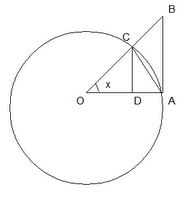## Thursday, August 31, 2006

### sin x < x < tan x for x in (0,π/2)

In today's blog, I will show how it is possible to use a unit circle to establish that if x is greater than 0 and less than π/2, then sin x is less than x which is less than tan x.

Theorem: if x is in (0,π/2), then sin x is less than x is less than tan xProof:

(1) Let O be a circle with radius = 1.

(2) Let ∠ CDO and ∠ BAO be right angles.

(3) Let x be the angle at ∠ COD

(4) We can see the following values apply to this diagram

cos x = adjacent/hypotenuse = OD/OC = OD/1 = OD

sin x = opposite/hypotenuse = CD/OC = CD/1 = CD

tan x = sin x/cos x = opposite/adjacent = AB/OA =AB/1 = AB

(5) Area of triangle OAC = (1/2)(base)(height) = (1/2)(OA)(CD) = (1/2)(1)(sin x) = (1/2)(sin x) [See Lemma 2, here for proof]

(6) Area of sector OAC = (1/2)(x)(radius)2 = (1/2)(x)(1)2 = (1/2)x [See Lemma 2, here for proof]

(7) Area of triangle OAB = (1/2)(base)(height) = (1/2)(OA)(AB) = (1/2)(1)(tan x) = (1/2)(tan x) [See Lemma 2, here for proof]

(8) By the diagram above, it is clear that triangle OAB is greater than sector OAC which is greater than triangle OAC.

(9) This then gives us that (1/2)(tan x) is greater than (1/2)(x) which is greater than (1/2)(sin x).

(10) Dividing all values by (1/2) gives us:

tan x is greater than x which is greater than sin x.

QED

ReferencesAnonymous said...

Thanks for the lesson. It helped me understand the squeezing theorem in my Calculus book.

UNC Greensboro StudentAnonymous said...

Area of triangle OCD is less than the sector's area

=> (1/2)*sin x *cos x <(1/2)*x

And then I was stuck...

I thought that the inequality could be written as

sin(2x) < (2x), 0< (2x) < (pi/2)

Assuming y = 2x,

sin y < y, 0< y < (pi/2)

Is this a valid proof? Or am I kidding myself?